## Example of Real Gas Calculation

This example will demonstrate how to calculate the compressibility of real gas in order to determine gas density and specific gravity at a specific condition.

Calculate the following based on the given condition:

1) Density of this gas under the reservoir conditions of 7,500psia and 220ºF,

2) Specific gravity of the gas.

Gas component is shown in Table 1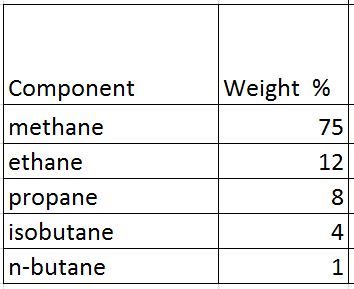Table 1 – Gas Component

Average density of air = 28.96 lb/cu-ft

## Solution

• Determine critical pressure and temperature of gas mixtures using Kay’s ruleTable 2 – Critical Pressure and Temperature

Note: critical pressure and temperature can be found from this link – https://www.drillingformulas.com/determine-compressibility-of-gases/

Pc’ = Σyipci = 660.5 psia

Tc’ = ΣyiTci = -46.2 F = -46.2 +460 F = 413.8 R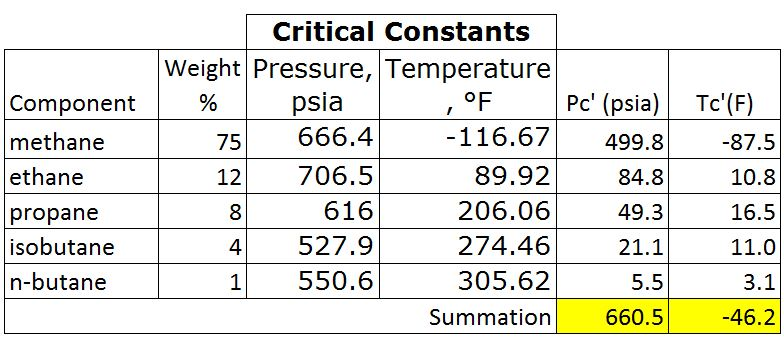Table 3 – Pc’ and Tr’ by Kay’s Rule

• Calculate Tr and Pr

Tr = T ÷Tc

Tr = (220+460) ÷ (-46.2+460)

Tr = 1.64

Note: temperature must be in Rankin.

Rankin = Fahrenheit + 460

For the critical temperature calculation, it can be converted the critical temperature from F to R before calculating Tc’. This will still give the same result.

Pr = P ÷ Pc

Pr = 7500 ÷ 660.5 = 11.4

• Read the compressibility factor (z) from the chart.

z = 1.22Figure 1-z-factor from the Standing and Katz Chart

• Calculate average molar mass

Average Molar Mass = Σyi×Mi = 22.1 lbTable 4 – Average Molar Mass of Gas

• Calculate density of gas from the equation below;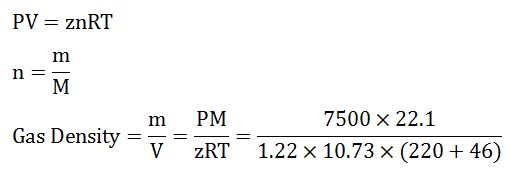Gas Density = 18.6 lb/cu-ft

• Calculate gas specific gravity from the equation below;

SG = Gas Density ÷ Air Density

SG = 18.6 ÷ 28.96

SG = 0.64

Summary:

Gas Density = 18.6 lb/cu-ft

SG = 0.64

We wish that this example will help you understand to determine z-factor and use it to calculate any related information.

References

Abhijit Y. Dandekar, 2013. Petroleum Reservoir Rock and Fluid Properties, Second Edition. 2 Edition. CRC Press.

L.P. Dake, 1983. Fundamentals of Reservoir Engineering, Volume 8 (Developments in Petroleum Science). New impression Edition. Elsevier Science.

Tarek Ahmed PhD PE, 2011. Advanced Reservoir Management and Engineering, Second Edition. 2 Edition. Gulf Professional Publishing.

## Lost Circulation and Well ControlLost circulation is a situation when drilling fluid losses downhole because formation(s) is fractured. There are three levels of lost circulation which are seepage loss, partial loss and total loss.Seepage loss is a situation when the mud volume loses into formation at very minimal and this will have no or little effect for a drilling operation.

Partial loss is a situation when some volume of drilling fluid loses into the well and you get some drilling mud volume back on surface. Not only do you lose the fluid volume, but you may have ballooning issue to deal. However, this type of fluid loss will not lead to well control situation because the total hydrostatic pressure does not decrease.

Total loss is the worst situation because there is no mud returning back to surface and the mud level will drop to any level down hole. Losing a lot of fluid into the well will directly affect hydrostatic pressure at the bottom. If you cannot keep the hole full, it might be a time when the hydrostatic pressure is less than the reservoir pressure. Eventually, a well control situation will be happened.

Additional information – What Cause Lost Circulation in Drilling Leading to a Well Control Situation

## Determine Bottom Hole Pressure from Wellhead Pressure in a Dry Gas Well

Gas behaves differently from fluid therefore you cannot use a simple hydrostatic formula to determine reservoir pressure. Gas is compressible but fluid is incompressible.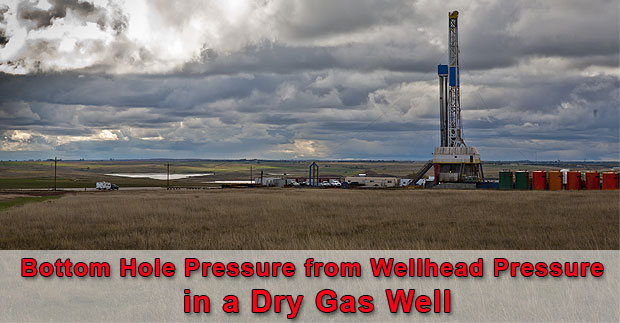The formula to determine the bottom hole pressure of dry gas well is shown below;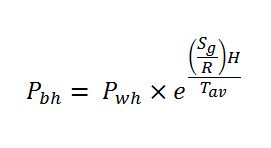Where; Pbh = bottom hole pressure in psia (absolute pressure)

Pwh = wellhead pressure in psia (absolute pressure)

H = true vertical depth of the well

Sg = specific gravity of gas

R = 53.36 ft-lb/lb-R (gas constant for API standard condition air)

Tav = average temperature in Rankin (Rankin = Fahrenheit + 460) Continue reading

## Learn about Maximum Surface Pressure in Well Control (MASP, MISICP and MAASP)

There are several terms/acronyms about maximum surface pressure in well control such as MASP, MISICP and MAASP. These terms sometimes confuses a lot of people hence this article will explain each term and demonstrate how to use it.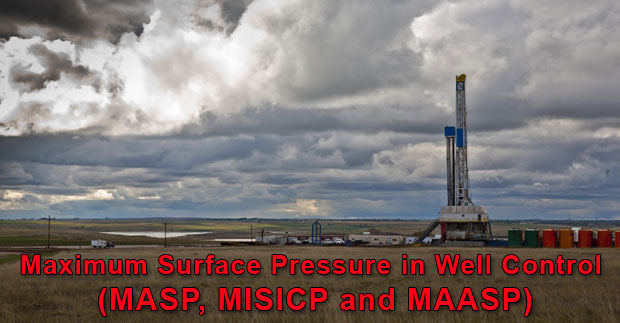## Leak Off Test (LOT)

The first factor you need to understand is Leak of test pressure (LOT). LOT is the surface pressure that breaks down formation at a casing shoe for each section of the well.

Leak off test pressure formula is listed below;

Leak off test pressure, psi = Surface pressure to break formation, psi + Hydrostatic pressure, psi

Typically, leak off test pressure is describe in equivalent mud density term therefore the formulas will be like this

Leak off test pressure, ppg = (Surface pressure to break formation, psi ÷ 0.052 ÷ shoe TVD, ft) + Mud weight, ppg Continue reading

## Fatality Drilling Rig Accident, Oilfield Worker was Knocked 6 feet to the floor

This is the oilfield incident resulting in our friend working on the rig got killed. It is very important that we need to heavily focus on the safety. Every year, people get injured due to several causes while working in the oilfield. For this case, there are several contributing factors the high pressure, line of fire, equipment inspection, etc. We want you to work safely for yourself and your family.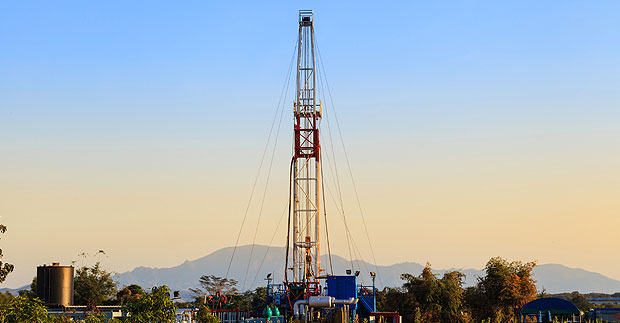A high-pressure gate valve that came off a leaky standpipe on a Cyclone rig north of Parachute early in the evening of Oct. 21 struck and killed Shane Hill, 34, of Grand Junction and blew his body 6 feet away from the valve, a state report says.